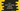# 5 different C++ programs to print one string without a semicolon## Introduction :

In this post, I will show you how we can print one string without semicolon in C++. In C++, we need to end one statement with a semicolon to complete it. But there are ways to execute one statement without a semicolon.

The idea is to put the statement inside a condition checker, i.e. if we put it inside an if, while, or switch, it will execute that statement and that statement will print the result.

### Method 1: Using while loop :

while loop takes one condition and executes its body if the condition is true. We can pass one print statement as the condition in a while loop like below :

``````#include <iostream>
using namespace std;

int main()
{
while (!(cout << "Hello World\n"))
{
}

return 0;
}``````

Here, we are checking the ! of the value that cout expression returns. This is because the loop will execute for infinite times if we don’t use the not operator. If you execute this program, it will print the “Hello World” line. We are keeping the body of the while loop empty because without a body we can’t execute one while loop.

### Method 2: Using a for loop :

It is similar to the while loop solution we did above. for loop takes three conditions. We can keep the first and the last one empty and add the print cout statement as the second one.

``````#include <iostream>
using namespace std;

int main()
{
for (; !(cout << "Hello World\n");)
{
}

return 0;
}``````

We are using one not here. Otherwise, it will run for an infinite time.

### Method 3: Using a ‘if’ block :

if checks for a condition before executing its body. We can place cout as its condition :

``````#include <iostream>
using namespace std;

int main()
{
if(cout << "Hello World\n")
{
}

return 0;
}``````

### Method 4: Using macros :

Macros are used to define a constant at the top level of a program. We can also create one macro as a cout expression and we can use that in other places like in a if condition.

Example program :

``````#include <iostream>
#define HELLO cout<<"Hello World\n"

using namespace std;

int main()
{
if(HELLO)
{
}

return 0;
}``````

The if block will execute the cout expression and print that Hello World string.

### Method 5: Using a switch block :

We can even use one switch block in a similar way. We can leave the body part empty. For example, the below example will print the Hello World string :

``````#include <iostream>
using namespace std;

int main()
{
switch (printf("Hello World\n"))
{
}

return 0;
}``````

We can’t use cout here because switch requires integral values and printf returns one integer, cout not.